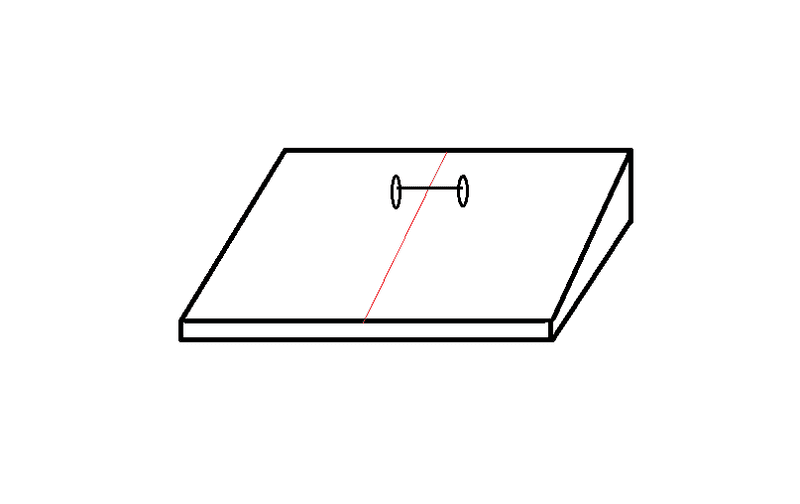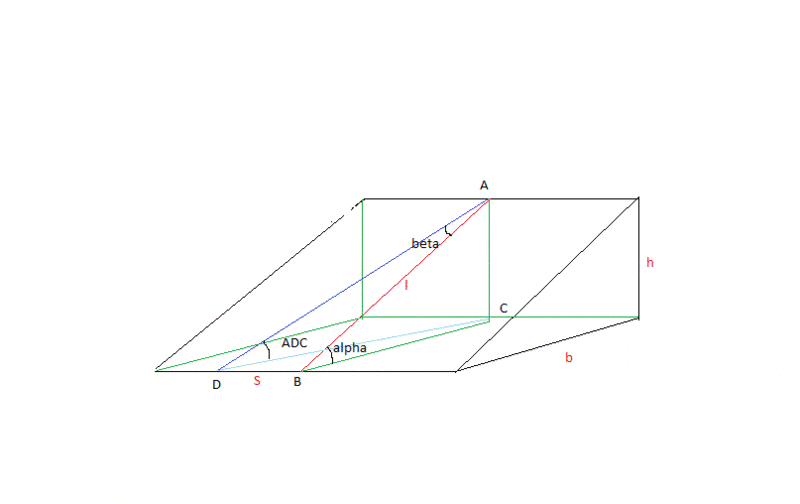# Falling at an angle

##### Emperor
Gold Member
1. The problem statement, all variables and given/known dataLet the angle of the block(slope) to be 30°.
Smallest height on diagram is 0m

Let the red line to divide the block by 2(when viewed from the top)
The two wheels are of equal diameter and is connected by a rod.
The total mass of two wheels + rod is 1kg.
Let the angle be measured from the red line.(At the image,the wheel system is 90° to the line)
1-Rotate the wheel to 0°.
It will not move.
2-Rotate to 90°
it will fall at maximum speed.
3- Rotate to 45°
will be slower than 90°.

So I am thinking of the relation between the angle and the speed of fall.

2. Relevant equations
$F=ma$
....

3. The attempt at a solution
Sorry I am at Gr.10. So I haven't studied vectors,forces or speeds at angles.
-Just curious-

#### Attachments

• 7.3 KB Views: 259

#### voko

A useful property here is the conservation of energy. When the system moves on the incline, its centre of mass gets lower. That means its potential energy is reduced. Which in turn means its kinetic energy is increased. Clearly when it goes "sideways" it needs to go a longer distance to gain the same amount of energy as compared with the case when it goes "straight".

Alternatively, you can look at the system so that your line of sight is always parallel to the axis of the system. Then you will see that it effectively rolls on an incline with some angle that depends on the "real" incline angle and "sideways" angle.

•1 person

##### Emperor
Gold Member
Thank you.
Any specific equations for these kinds of "incline" things?

#### voko

I think you could try figuring out the "effective" incline angle. This is pure geometry.

•1 person

##### Emperor
Gold Member
I don't understand what you mean by the axis of the system.
Anyway,I want to find the relationship.
So I have to make an equation relating incline angle and wheel angle.

#### voko

Let the top point of the red line be A. The lowest point of the red line is B. The point on the ground under A is C, so ABC is a right triangle, and the angle ABC is $\alpha$.

The system starts at A and rolls down the incline at some angle with the red line and it touches the ground at D. The angle DAB is the "sideways" angle $\beta$. The "effective" incline angle is ADC. Express angle ADC in terms of $\alpha$ and $\beta$.

•1 person

##### Emperor
Gold Member
Let the top point of the red line be A. The lowest point of the red line is B. The point on the ground under A is C, so ABC is a right triangle, and the angle ABC is $\alpha$.

The system starts at A and rolls down the incline at some angle with the red line and it touches the ground at D. The angle DAB is the "sideways" angle $\beta$. The "effective" incline angle is ADC. Express angle ADC in terms of $\alpha$ and $\beta$.
Isn't D the same as B?I said in the OP that lowest point of the red line and the ground has a distance of 0m

#### voko

Isn't D the same as B?
How? The system rolls away from the red line, so it cannot end up at B.

I said in the OP that lowest point of the red line and the ground has a distance of 0m
Do you think I indicated otherwise?

##### Emperor
Gold Member
How? The system rolls away from the red line, so it cannot end up at B.
Oh sorry.I thought B as the point of ground.
Ok.Its a point on the red line and D is a point on ground(Where it touches)

How's that going to relate wheel angle and speed?

#### voko

Oh sorry.I thought B as the point of ground.
Ok.Its a point on the red line and D is a point on ground(Where it touches)
I do not think you understood me. Points B, C, D are all on the ground. Point B in particular is where the red line touches the ground. Point D is where the rolling system touches the ground. These two points can also be said to lie on the edge of the incline.

How's that going to relate wheel angle and speed?
I do not know what the "wheel angle" is and I would like you to answer my question about angle ADC first, anyway.

•1 person

##### Emperor
Gold Member
Let the top point of the red line be A. The lowest point of the red line is B. The point on the ground under A is C, so ABC is a right triangle, and the angle ABC is $\alpha$.

The system starts at A and rolls down the incline at some angle with the red line and it touches the ground at D. The angle DAB is the "sideways" angle $\beta$. The "effective" incline angle is ADC. Express angle ADC in terms of $\alpha$ and $\beta$.
I would like you to answer my question about angle ADC first, anyway.
That seems to be a 3d problem.How am I going to do that?I haven't studied that yet.Can you give me a hint?

#### voko

You solve a 3D problem by disassembling it into 2D subproblems.

In this case, you have three triangles: ABC, ABD, and ADC. These are right triangles and they are all related to each other in some way. Triangle ABD and triangle ADC share hypotenuse AD. Triangle ABC and triangle ADC share cathetus AC. This is enough to express angle ADC via angle ABC ($\alpha$) and angle DAB ($\beta$), it takes just a bit of trigonometry.

•1 person

##### Emperor
Gold Member
Is it OK to include other variables like BD,CB and AC?

#### voko

There is an answer that only has $\alpha$ and $\beta$. But we can discuss your approach even if you do not have that answer yet. Show what you have so far.

##### Emperor
Gold Member
OK.I've got
$\alpha=tan^{-1}(\frac{h}{b})$
$\beta=tan^{-1}(\frac{S}{\sqrt{h^2+b^2}})$
$ADC=tan^{-1}(\frac{h}{\sqrt{s^2+b^2}})$

Not sure how to link $\alpha$,$\beta$ and ADC in one equationEDIT:Latex problem.How do I fix that?

#### Attachments

• 8.9 KB Views: 220
Last edited:

#### voko

You meant those:
$\alpha=tan^{-1}(\frac{h}{b})$
$\beta=tan^{-1}(\frac{S}{\sqrt{h^2+b^2}})$
$ADC=tan^{-1}(\frac{h}{\sqrt{s^2+b^2}})$

(one closing curly brace was missing)

##### Emperor
Gold Member
You meant those:
$\alpha=tan^{-1}(\frac{h}{b})$
$\beta=tan^{-1}(\frac{S}{\sqrt{h^2+b^2}})$
$ADC=tan^{-1}(\frac{h}{\sqrt{s^2+b^2}})$

(one closing curly brace was missing)
Yeah,it's that.
How do I link those three together?#### voko

As I said:
Triangle ABD and triangle ADC share hypotenuse AD. Triangle ABC and triangle ADC share cathetus AC.
So, can you express AD via AB and $\beta$? Then, can you express AB via AC and $\alpha$? And, finally, find the ratio of AC with AD?

•1 person

##### Emperor
Gold Member
So, can you express AD via AB and $\beta$?
$AD=\frac{AB}{cos\beta}$

Then, can you express AB via AC and $\alpha$?
$AB=\frac{AC}{sin\alpha}$

And, finally, find the ratio of AC with AD?
How do I do that?I don't understand what you said.

#### voko

You are required to find a certain angle, let's call it $\gamma$. Is it related in any way with $$AC \over AD$$ ?

### The Physics Forums Way

We Value Quality
• Topics based on mainstream science
• Proper English grammar and spelling
We Value Civility
• Positive and compassionate attitudes
• Patience while debating
We Value Productivity
• Disciplined to remain on-topic
• Recognition of own weaknesses
• Solo and co-op problem solving